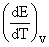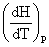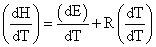# Heat Capacity Assignment Help

## 7.5 Heat Capacity

The quantity of heat required to raise the temperature of the system by one degree.

Specific Heat

The amount of heat required to raise the temperature of 1 gm of a substance by 1 degree is called specific heat.

Molar Specific Heat

The amount of heat required to raise the temperature of 1 mole of a substance by 1 degree is called molar specific heat.

At constant volume qV = DEMolar sp. heat at constant volume CV =At constant pressure qp = DH

Molar sp. heat at constant pressure

Cp =H = E + PV

H = E + RT (for one mole of ideal gas)CP = CV + R

CP – CV = R